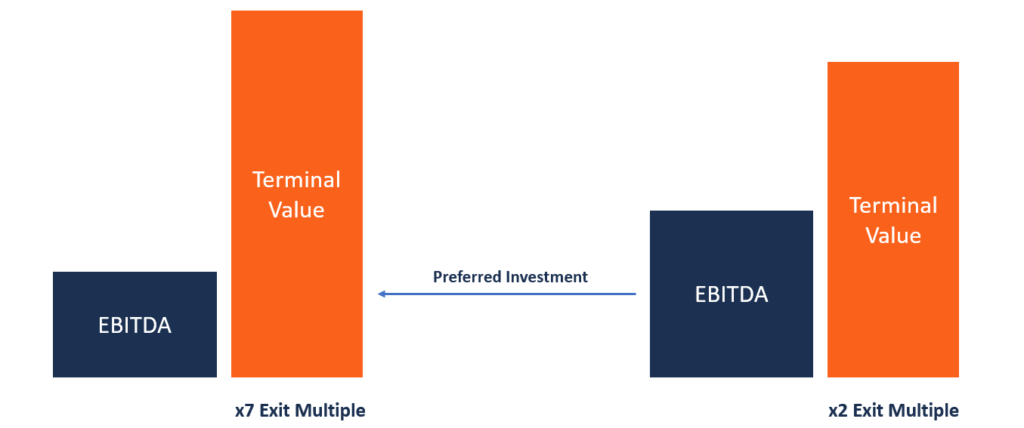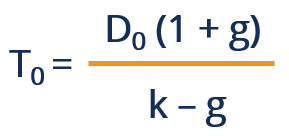# Exit Multiple

The value of a business will be determined at the end of a projection period

## What is Exit Multiple?

Exit multiple is one of the methods used to calculate the terminal value of the free cash flows of a business. The method assumes that the value of a business will be determined at the end of a projected period, based on the existing public market valuations. The most commonly used multiples are EV/EBITDA and EV/EBIT.Analysts use exit multiples to estimate the value of a company by multiplying financial metrics such as EBIT and EBITDA by a factor that is similar to that of recently acquired companies. Exit multiple is sometimes referred to as terminal exit value.

### What is Terminal Value?

Terminal value refers to the value at a future point in time of all future cash flows of a company when the growth rate is expected to be consistent and stable. However, terminal value limits the cash flow projections to a several-years period. Usually, predicting the value of a business beyond such a period is often impossible, and it exposes such a process to a variety of risks that question the validity of the forecast.

Terminal value addresses such limitations by allowing the inclusion of future cash flow values beyond the projection period while mitigating any issues that may arise from using the values of such cash flows.

### Using Discounted Cash Flows Method to Determine Terminal Value

When estimating a company’s cash flows in the future, analysts use financial models such as the discounted cash flow (DCF) method combined with certain assumptions to arrive at the value of the business. The DCF method assumes that the asset value equals the future cash flows generated by that asset.

The discounted cash flow method comprises two main components, i.e., the forecast period and terminal value. The forecast period is used when estimating the value of a company or asset for a period of about five years. Using the forecast period to determine the value of a company for a period exceeding five years will cast into doubt the accuracy of the valuation obtained. Using terminal value to find the value of a company or asset attempts to solve the issues that emerge when using the forecast period.

### Calculating Terminal Value Using Exit Multiple

The exit multiple assumes that the market multiple basis is a fair method of valuing a business. The value of the business is obtained by multiplying financial metrics such as EBITDA or EBIT by a factor that is common to comparable companies that were recently acquired. An appropriate range of multiples can be generated by looking at recent comparable acquisitions in the public market.

The multiple obtained is then multiplied by the projected EBIT or EBITDA in year N (final year of projection period) to give the future value at the end of year N. The future value (also known as terminal value) is then discounted by a factor equal to the number of years in the projection period.

The value obtained is then added to the present value of the free cash flows to obtain the implied enterprise value. For cyclical businesses where earnings fluctuate according to variations in the economy, we use the average EBITDA or EBIT during the course of the specific cyclical rather than the amount in year N in the projection period.

This means that an industry multiple is applied rather than applying a current multiple to take into account the cyclical variations of earnings. If analysts used a current multiple, the valuation would be affected by economic cycles.

### Perpetual Growth Method

The perpetual growth method is an alternative to the exit multiple method, and it accounts for the free cash flows of a business that grow at a steady rate in perpetuity. It assumes that cash will grow at a stable rate forever starting from a specific point in the future.

While it is nearly impossible for a company to grow at the same rate for an infinite period in the future, the perpetual growth method is preferred to calculating the terminal value because it is based on the historical performance of the company.

Compared to the exit multiple method, the perpetual growth method generates a higher terminal value. The formula for calculating the terminal value using the perpetual growth method is as follows:Where:

• D0 represents the cash flows at a future period that is prior to N+1 or towards the end of period N.
• k represents the discount rate
• g represents the constant growth rate

CFI is the official provider of the global Financial Modeling & Valuation Analyst (FMVA)™ certification program, designed to help anyone become a world-class financial analyst. To keep advancing your career, the additional CFI resources below will be useful:

• Comparable Company Analysis
• EBIT vs EBITDA
• Entry Multiple
• Types of Valuation Multiples

### Financial Analyst Training

Get world-class financial training with CFI’s online certified financial analyst training program!

Gain the confidence you need to move up the ladder in a high powered corporate finance career path.

Learn financial modeling and valuation in Excel the easy way, with step-by-step training.